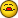# Excel Subtotals

A

#### Austin

Good Morning,

I am working with some code that exports from Access to Excel and then
subtotals in Excel. Like the code below:

With xlTop.Range("A")
.Subtotal GroupBy:=1, Function:=xlSum, TotalList:=Array(3, 4, 5, 6, 7,
8, 9, 10, 11, 12, 13, 14, 15), _
Replace:=True, PageBreaks:=False, SummaryBelowData:=xlSummaryBelow
End With

The problem is that the width of the recordset being subtotaled on can
change (it will not necessarily be 15 columns wide). Is there a way to make
this range dynamic?

Thanks,

Austin

J

#### Joel

Try thjis

Sub test()

Dim MyArray() As Variant

StartCol = 3
EndCol = 15

ReDim MyArray(0 To (EndCol - StartCol))
For i = StartCol To EndCol
MyArray(i - StartCol) = i
Next i

Set sht = Sheets("Sheet1")

With sht.Range("A")
.Subtotal GroupBy:=1, Function:=xlSum, _
TotalList:=MyArray, _
Replace:=True, _
PageBreaks:=False, _
SummaryBelowData:=xlSummaryBelow
End With
End Sub

A

#### Austin

Perfect, thanks Joel

Joel said:
Try thjis

Sub test()

Dim MyArray() As Variant

StartCol = 3
EndCol = 15

ReDim MyArray(0 To (EndCol - StartCol))
For i = StartCol To EndCol
MyArray(i - StartCol) = i
Next i

Set sht = Sheets("Sheet1")

With sht.Range("A")
.Subtotal GroupBy:=1, Function:=xlSum, _
TotalList:=MyArray, _
Replace:=True, _
PageBreaks:=False, _
SummaryBelowData:=xlSummaryBelow
End With
End Sub